SLVSE46A November   2017  – January 2018

PRODUCTION DATA.

1. Features
2. Applications
3. Description
4. Revision History
5. Pin Configuration and Functions
6. Specifications
7. Detailed Description
1. 7.1 Overview
2. 7.2 Functional Block Diagram
3. 7.3 Feature Description
4. 7.4 Device Functional Modes
5. 7.5 Programming
1. 7.5.1 I2C Bus Operation
6. 7.6 Register Map
1. 7.6.1 Register Description
8. Applications and Implementation
1. 8.1 Application Information
2. 8.2 Typical Application
9. Power Supply Recommendations
10. 10Layout
11. 11Device and Documentation Support
12. 12Mechanical, Packaging, and Orderable Information

#### Package Options

Refer to the PDF data sheet for device specific package drawings

• RSN|32

#### 8.2.3 Application CurvesCL = 1 nF VGH = 14 V VGL1 = –15 V VGL2 = –15 V
Figure 239. Peak Output Current (GCKx)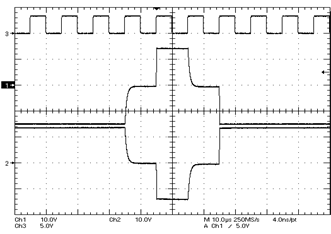CL = 1 nF VGH = 14 V CH1 = GCK1 RCS = 1 kΩ VGL1 = –15 V CH2 = GCK11 VGL2 = –15 V CH3 = LN_CLK
Figure 241. Charge-SharingCL = 8 pF VGH = 14 V VGL1 = –15 V VGL2 = –15 V
Figure 243. Rise Time (GCKx)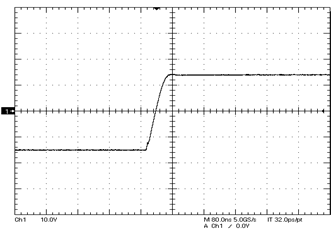CL = 1 nF VGH = 14 V VGL1 = –15 V VGL2 = –15 V
Figure 245. Rise Time (GCKx)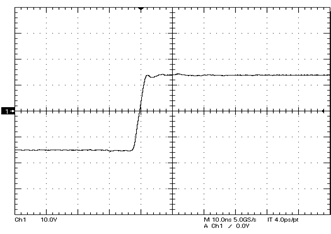CL = 8 pF VGH = 14 V VGL1 = –15 V VGL2 = –15 V
Figure 247. Rise Time (GSPx,GGPx,GCP)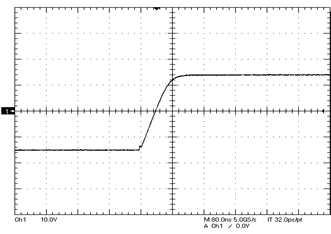CL = 1 nF VGH = 14 V VGL1 = –15 V VGL2 = –15 V
Figure 249. Rise Time (GSPx,GGPx,GCP)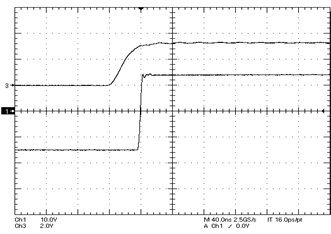PLL bypassed VGH = 14 V CH1 = GCK1 CL = 8 pF VGL1 = –15 V CH3 = LN_CLK VGL2 = –15 V
Figure 251. Propagation Delay tPLH (GCKx)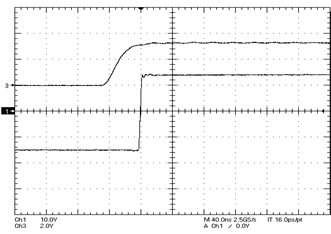PLL bypassed VGH = 14 V CH1 = GGP2 CL = 8 pF VGL1 = –15 V CH3 = LN_CLK VGL2 = –15 V
Figure 253. Propagation Delay tPLH (GSPx,GGPx,GCP)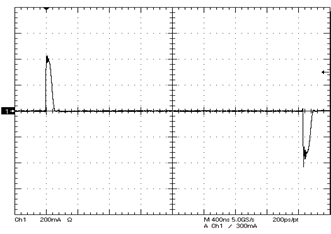CL = 1 nF VGH = 14 V VGL1 = –15 V VGL2 = –15 V
Figure 240. Peak Output Current (GSPx,GGPx,GCP)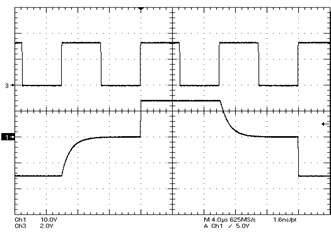CL = 1 nF VGH = 14 V CH1 = GCK1 RGVS = 1 kΩ VGL1 = –15 V CH3 = LN_CLK VGL2 = –15 V
Figure 242. Gate-Voltage Shaping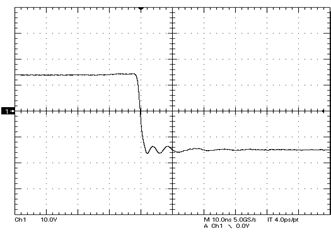CL = 8 pF VGH = 14 V VGL1 = –15 V VGL2 = –15 V
Figure 244. Fall Time (GCKx)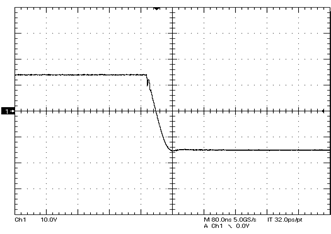CL = 1 nF VGH = 14 V 90% and 10% VGL1 = –15 V VGL2 = –15 V
Figure 246. Fall Time (GCKx)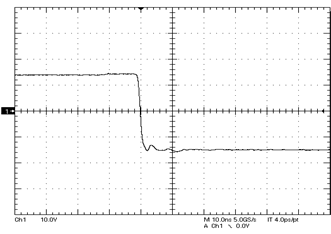CL = 8 pF VGH = 14 V VGL1 = –15 V VGL2 = –15 V
Figure 248. Fall Time (GSPx,GGPx,GCP)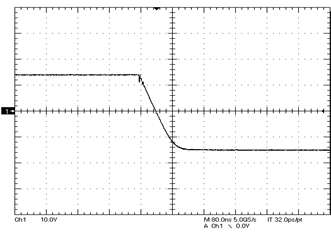CL = 1 nF VGH = 14 V VGL1 = –15 V VGL2 = –15 V
Figure 250. Fall Time (GSPx,GGPx,GCP)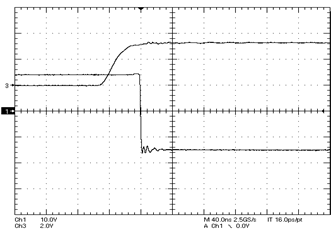PLL bypassed VGH = 14 V CH1 = GCK1 CL = 8 pF VGL1 = –15 V CH3 = LN_CLK VGL2 = –15 V
Figure 252. Propagation Delay tPHL (GCKx)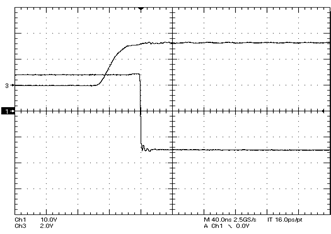PLL bypassed VGH = 14 V CH1 = GGP2 CL = 8 pF VGL1 = –15 V CH3 = LN_CLK VGL2 = –15 V
Figure 254. Propagation Delay tPHL (GSPx,GGPx,GCP)